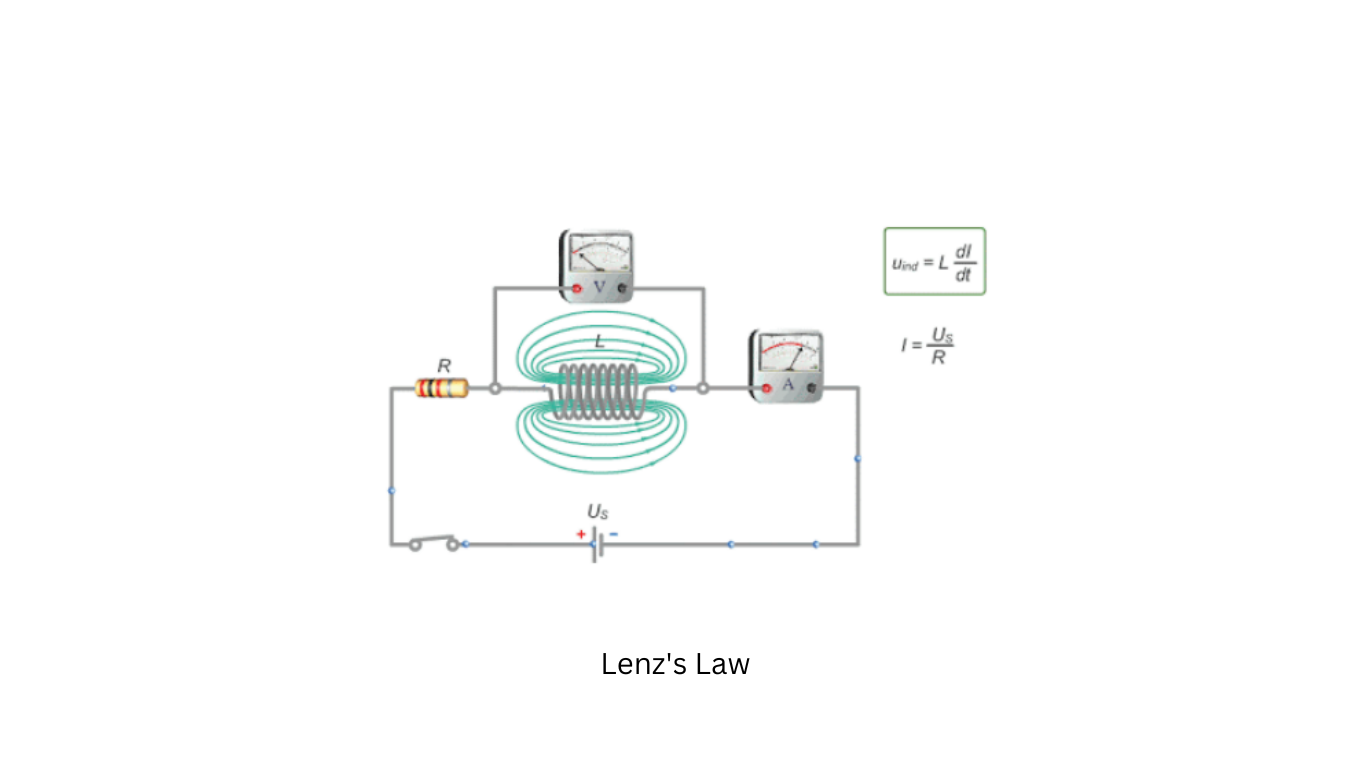## Lenz’s Law

Lenz’s Law is a fundamental principle in electromagnetism that describes the relationship between a changing magnetic field and the induced electromotive force (EMF) or voltage in a nearby conductor, typically a coil of wire. This law is a consequence of Faraday’s law of electromagnetic induction.Lenz’s Law can be stated as follows:

“When there is a change in the magnetic flux through a closed circuit, an electromotive force (EMF) is induced in the circuit, and the direction of this induced EMF opposes the change in magnetic flux that produced it.”

In simpler terms, Lenz’s Law tells us that when the magnetic field around a coil or conductor changes, it induces a voltage in such a way that it creates a current that opposes the change in the magnetic field. This is often summarized as “Nature abhors a change in flux.”

For example, if you were to increase the current passing through a coil, it would generate a stronger magnetic field. However, Lenz’s Law states that this change in magnetic field induces a voltage that opposes the increase in current. Conversely, if you were to decrease the current, the induced voltage would act in a way to oppose the decrease in current.

This law is essential in understanding various electromagnetic phenomena, including the behavior of transformers, generators, and inductors, as well as explaining why a moving magnet near a coil generates an electric current. It ensures that energy is conserved and that the system resists abrupt changes in magnetic flux, ultimately maintaining stability.

Share.
error: Content is protected !!